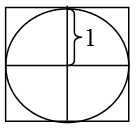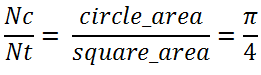## Program to calculate pie, C/C++ Programming

Assignment Help:

This problem familiarizes you with using random numbers in C++. The program is to compute a good approximation of π using a simulation method called "Monte Carlo". The following figure shows a circle of radius 1, centered at the origin and circumscribed by a square.Imagine that this is a dartboard and that you are tossing darts at it randomly. With enough darts, the ratio of darts in the circle (Nc) to total darts thrown (Nt) is approximately equal the ratio between the area of the circle (circle_area = π(1)2) and the area of the square (square_area = 4) i.e.Therefore, 4=NC/NT . We can simplify the math by only considering the first quadrant, calculating the ratio of the number of darts landed on the first quadrant over the number of darts landed on the top right square's area.

Use the Euclidean distance formula, to check if a dart is landed inside the first quadrant. Maintain account of the number of darts that end up in the first quadrant of the unit circle.

Now use your loop to build a π-calculating function. The function should take one argument specifying the number of dart throws to run. It should return the decimal value of π, using the technique outlined above. You should get pretty good results for around 5,000,000 dart throws! Isn't this neat?

#### How do you know that your class requires virtual destructor, How do you kn...

How do you know that your class requires a virtual destructor? A: If at least your class has one virtual function, you must make a destructor for this class virtual. It will let

#### Mathematical statements, Mathematical Statements and assignments    Wi...

Mathematical Statements and assignments    Within C we can directly load up the variable from within the program using the mathematical expression equates (=) e.g.   a= 'h'

#### Poop, hwat is the area fsdjlakl;aeklfjtealrtl;gka

hwat is the area fsdjlakl;aeklfjtealrtl;gka

#### #superASC2 SRTING COST, A string S is said to be "Super ASCII", if it conta...

A string S is said to be "Super ASCII", if it contains the character frequency equal to their ascii values. String will contain only lower case alphabets (''a''-''z'') and the asci

#### Explain about evaluation of expression in c language, Explain about Evaluat...

Explain about Evaluation of Expression in c language? An Expressions are evaluated using an assignment statement of the form: variable = expression; The Variable is any v

#### Board coloring, coloring of elements in matrix form inm particular matrix.t...

coloring of elements in matrix form inm particular matrix.the color should not match with another color.

#### Fibonacci series, draw the flow chart to print the fibonacci series upto n ...

draw the flow chart to print the fibonacci series upto n th terms

#### Car rental system, Car Rental System This system tracks cars in a rental co...

Car Rental System This system tracks cars in a rental company. Each car has a number (assume its plate number), type (small car, four wheel car), and status (rented, available). T

#### Sentinel controlled loop, develop an algorithm using pseudocode for computi...

develop an algorithm using pseudocode for computing cos(x) and sin(x). use a sentinel controlled while loop. use the series definition of e^+-jx

#### C program for find even & odd no.s in the array , C Program for FIND EVEN &...

C Program for FIND EVEN & ODD NO.S IN THE ARRAY #include stdio.h> #include string.h> #include conio.h> void main() {           int i=0,j=0, l_e,l_o;